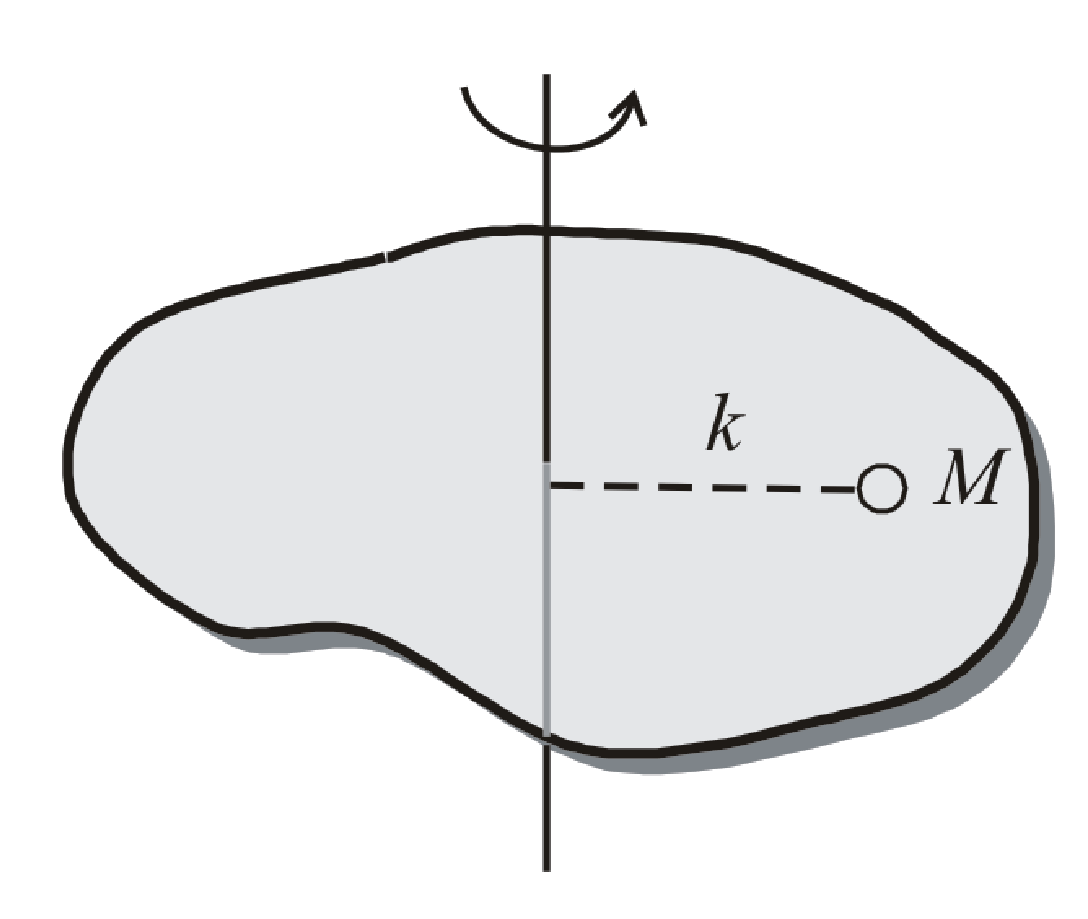BodhiAI
Oct. 31, 2019

Radius of gyration of a body about a given axis is the perpendicular distance of a point from the axis, where whole mass of the body were concentrated, the body shall have the same moment of inertia as it has with the actual distribution of mass.

I = Mk2 orFrom the definition of radius of gyrationI = Mk2

(i) Radius of gyration (k) depends on shape and size of the body, position and configuration of the axis of rotation, distribution of mass of the body w.r.t. the axis of rotation.

(ii) Radius of gyration (k) does not depends on the mass of body.

(iii) Dimension : [M0L1T0]

(iv) S.I. Unit :Metre

Example :In case of a disc rotating about an axis through its centre of mass and perpendicular to its plane.